Next: 11.2 Convergence Tests Up: 11. Infinite Series Previous: 11. Infinite Series   Index

# 11.1 Infinite Series

11.1   Definition (Series operator.) If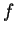is a complex sequence, we define a new sequence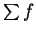by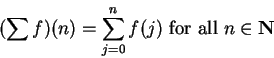or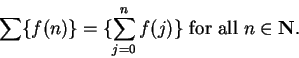We use variations, such as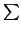is actually a function that maps complex sequences to complex sequences. We callthe series corresponding to.

11.2   Remark. If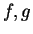are complex sequences and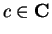, then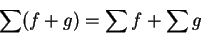andsince for all,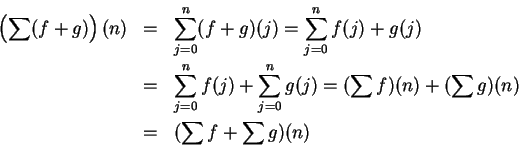and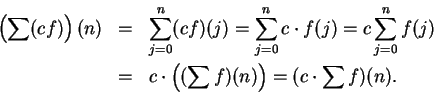11.3   Examples. If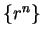is a geometric sequence, then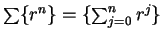is a sequence we have been calling a geometric series. If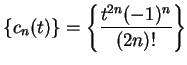, thenis the sequence for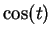that we studied in the last chapter.

11.4   Definition (Summable sequence.) A complex sequenceis summable if and only if the series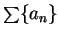is convergent. Ifis summable, we denote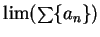by. We callthe sum of the series.

11.5   Example. Ifand, then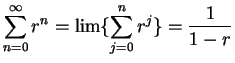.

11.6   Example (Harmonic series.) The seriesis called the harmonic series, and is denoted by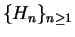. Thus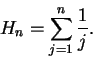We will show thatdiverges; i.e., the sequence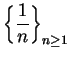is not summable. For all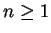, we have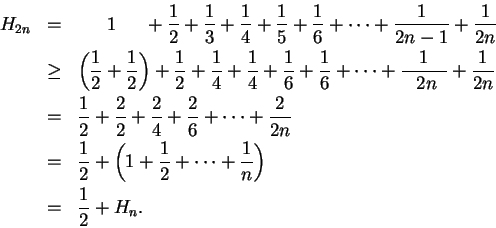From the relation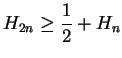, we have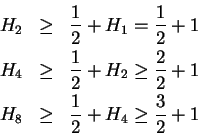and (by induction),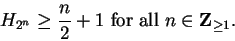Hence,is not bounded, and thus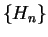diverges; i.e.,is not summable.

11.7   Theorem (Sum theorem for series.) Letbe summable sequences and let. Then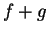and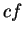are summable, andIfis not summable, and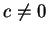, thenis not summable.

Proof: The proof is left to you.

11.8   Exercise. Letbe summable sequences. Show thatis summable and that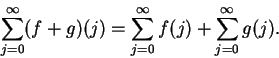11.9   Example. The product of two summable sequences is not necessarily summable. If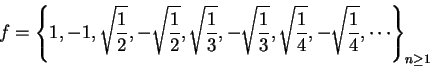then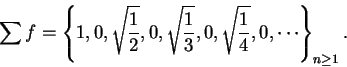This is a null sequence, sois summable and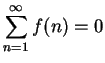. However,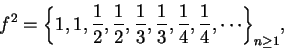so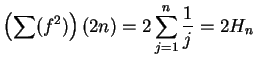. Thus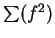is unbounded and hence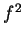is not summable.

11.10   Theorem. Every summable sequence is a null sequence. [The converse is not true. The harmonic series provides a counterexample.]

Proof: Letbe a summable sequence. Then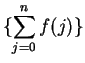converges to a limit, and by the translation theoremalso. Hence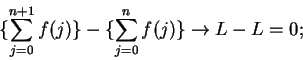i.e.,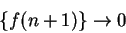and it follows thatis a null sequence.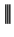Next: 11.2 Convergence Tests Up: 11. Infinite Series Previous: 11. Infinite Series   Index